## Apophenia

Databases

These are convenience functions to handle interaction with SQLite or mySQL/mariaDB. They open one and only one database, and handle most of the interaction therewith for you.

You will probably first use apop_text_to_db to pull data into the database, then apop_query to clean the data in the database, and finally apop_query_to_data to pull some subset of the data out for analysis.

• In all cases, your query may be in printf form. For example:
char tabname[] = "demographics";
char colname[] = "heights";
int min_height = 175;
apop_query("select %s from %s where %s > %i", colname, tabname, colname, min_height);

See the Database moments (plus pow()!) section below for not-SQL-standard math functions that you can use when sending queries from Apophenia, such as pow, stddev, or sqrt.

• apop_text_to_db : Read a text file on disk into the database. Data analysis projects often start with a call to this.
• apop_data_print : If you include the argument .output_type='d', this prints your apop_data set to the database.
• apop_query : Manipulate the database, return nothing (e.g., insert rows or create table).
• apop_db_open : Optional, for when you want to use a database on disk.
• apop_db_close : A useful (and in some cases, optional) companion to apop_db_open.
• apop_table_exists : Check to make sure you aren't reinventing or destroying data. Also, a clean way to drop a table.
• Apophenia reserves the right to insert temp tables into the opened database. They will all have names beginning with apop_, so the reader is advised to not generate tables with such names, and is free to ignore or delete any such tables that turn up.
• If you need to deal with two databases, use SQL's attach database. By default with SQLite, Apophenia opens an in-memory database handle. It is a sensible workflow to use the faster in-memory database as the primary database, and then attach an on-disk database to read in data and write final output tables.

# Extracting data from the database

• apop_db_to_crosstab : take up to three columns in the database (row, column, value) and produce a table of values.
• apop_query_to_data
• apop_query_to_float
• apop_query_to_mixed_data
• apop_query_to_text
• apop_query_to_vector

# Writing data to the database

See the print functions at Legible output. E.g.

apop_data_print(yourdata, .output_type='d', .output_name="dbtab");

# Command-line utilities

A few functions have proven to be useful enough to be worth breaking out into their own programs, for use in scripts or other data analysis from the command line:

• The apop_text_to_db command line utility is a wrapper for the apop_text_to_db command.
• The apop_db_to_crosstab function is a wrapper for the apop_db_to_crosstab function.

# Database moments (plus pow()!)

SQLite lets users define new functions for use in queries, and Apophenia uses this facility to define a few common functions.

• select ran() from table will produce a new random number between zero and one for every row of the input table, using gsl_rng_uniform.
• The SQL standard includes the count(x) and avg(x) aggregators, but statisticians are usually interested in higher moments as well—at least the variance. Therefore, SQL queries using the Apophenia library may include any of these moments:
select count(x), stddev(x), avg(x), var(x), variance(x), skew(x), kurt(x), kurtosis(x),
std(x), stddev_samp(x), stddev_pop(x), var_samp(x), var_pop(x)
from table
group by whatever

var and variance; kurt and kurtosis do the same thing; choose the one that sounds better to you. Kurtosis is the fourth central moment by itself, not adjusted by subtracting three or dividing by variance squared. var, var_samp, stddev and stddev_samp give sample variance/standard deviation; variance, var_pop, std and stddev_pop give population standard deviation. The plethora of variants are for mySQL compatibility.

• The var/skew/kurtosis functions calculate sample moments. If you want the second population moment, multiply the variance by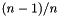; for the third population moment, multiply the skew by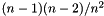. The equation for the unbiased sample kurtosis as calculated in Appendix M of Modeling with Data is not quite as easy to adjust.
• Also provided: wrapper functions for standard math library functions—sqrt(x), pow(x,y), exp(x), log(x), and trig functions. They call the standard math library function of the same name to calculate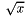,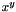,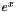,,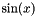,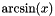, et cetera. For example:
select sqrt(x), pow(x,0.5), exp(x), log(x), log10(x),
sin(x), cos(x), tan(x), asin(x), acos(x), atan(x)
from table
• The ran() function calls gsl_rng_uniform to produce a uniform draw between zero and one. It uses the stock of RNGs from apop_rng_get_thread.

Here is a test script using many of the above.

#include <apop.h>
#define Diff(L, R) assert(fabs((L)-(R)<1e-4));
#define Diff2(L, R) assert(fabs((L)-(R)<1e-3));
#define getrow(rowname) apop_data_get(row, .colname=#rowname)
double test_all(apop_data *row){
Diff(gsl_pow_2(getrow(root)), getrow(rr))
Diff(getrow(ln), getrow(L10)*log(10))
Diff(getrow(rr), getrow(rragain))
Diff(getrow(one), 1)
return 0;
}
int main(){
apop_opts.db_engine='s'; //SQLite only.
//create a table with two rows.
//We didn't explicitly open a db with apop_db_open,
//so this will be an in-memory SQLite db.
apop_query("create table a(b); "
"insert into a values(1); "
"insert into a values(1); "
"create table randoms as "
"select ran() as rr "
/* join to create 2^13=8192 rows*/
"from a,a,a,a,a,a,a,a,a,a,a,a,a;");
apop_data *d = apop_query_to_data(
"select rr, sqrt(rr) as root, "
"log(rr) as ln, log10(rr) as L10, "
"exp(log(rr)) as rragain, "
"pow(sin(rr),2)+pow(cos(rr),2) as one "
"from randoms");
apop_map(d, .fn_r=test_all);
//the pop variance of a Uniform[0,1]=1/12; kurtosis=1/80.
Apop_col_tv(d, "rr", rrow);
Diff(apop_var(rrow)*8191./8192., 1/12. );
Diff(apop_vector_kurtosis(rrow)*8191./8192., 1/80.);//approx.
Diff(apop_query_to_float("select stddev(rr) from randoms"),
sqrt(1/12.)*8192./8191);
//compare the std dev of a uniform as reported by the
//database routine, the matrix routine, and math.
apop_query("create table atab (a numeric)");
for (int i=0; i< 2e5; i++)
apop_query("insert into atab values(ran())");
apop_query("create table powa as "
"select a, pow(a, 2) as sq, pow(a, 0.5) as sqrt "
"from atab");
double db_pop_stddev = apop_query_to_float("select stddev_pop(a) from powa");
d = apop_query_to_data("select * from powa");
//get the full covariance matrix, but just use the (0,0)th elmt.
double matrix_pop_stddev = sqrt(apop_data_get(cov)*(d->matrix->size1/(d->matrix->size1-1.)));
Diff(db_pop_stddev, matrix_pop_stddev);
double actual_stddev = sqrt(2*gsl_pow_3(.5)/3);
Diff2(db_pop_stddev, actual_stddev);
float sq_mean = apop_query_to_float("select avg(sq) from powa");
float actual_sq_mean = 1./3;
Diff2(sq_mean, actual_sq_mean);
float sqrt_mean = apop_query_to_float("select avg(sqrt) from powa");
float actual_sqrt_mean = 2./3;
Diff2(sqrt_mean, actual_sqrt_mean);
}